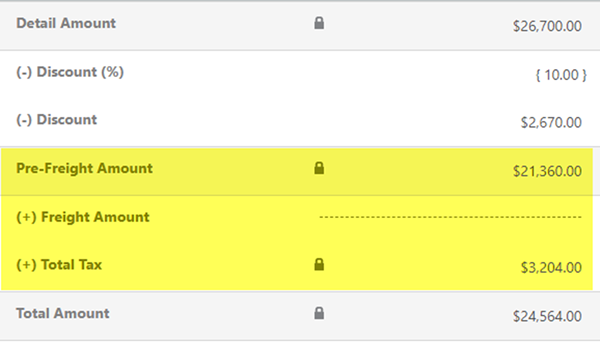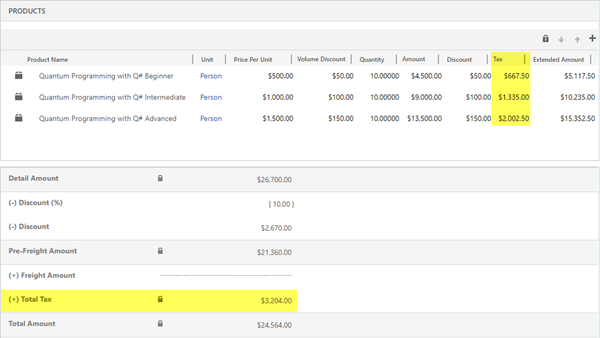# Tax and Discounts on Quotes/Orders in Dynamics 365 - Part 3 of 3

### Colin Maitland, 07 September 2018

In the third of this three-part series I will describe the second of two approaches that might be used for correctly calculating Total Tax on Quotes/Orders that have a Discount applied to the Quote/Order when it is desired that the calculation of Total Tax should take the Discount into consideration.

If there is a need for Tax to be applied at the Quote/Order Product level, then the following configuration and customisations might be used.

Step 1: Disable the Microsoft Dynamics 365 System Pricing Calculation. Refer to https://msdn.microsoft.com/en-us/library/dn817885.aspx. IMPORTANT NOTE: Disabling this option will require you to then provide custom programming to calculate all the previously system calculated pricing fields for Opportunities, Quotes Orders and Invoices.

If the same Tax Rate is always used for each of the Quote/Order Products, e.g. 15%, then:

Step 2: Override the system calculated Total Tax field on the Quote/Order to use one of the following calculations:

• If Total Tax includes tax on the Freight Amount, then Total Tax = Tax portion of (Pre-Freight Amount + Freight Amount).

• If Total Tax does not include tax on the Freight Amount, then Total Tax = Tax portion of (Pre-Freight Amount); e.g. 15% of \$21,360.00 = \$3,204.00.If a different Tax Rate might be used for each of the Quote/Order Products, e.g. 15% for some, and 0% for others such as might be the case with the purchase of Gift Voucher, then the following calculations should be used:

Step 2: Calculate the Quote/Order total Discount as a Percent as follows:

• Total Discount as a Percent = (Detail Amount – Pre-Freight Amount) / Detail Amount; e.g. (\$26,700.00 - \$21,360.00) / \$2,670.00 = \$5,340.00 / \$26,700.00 = 20%.

Step 3: Override the system calculated Total Tax field on the Quote/Order to use one of the following calculations:

• If Total Tax includes tax on the Freight Amount, then Total Tax = (Sum of related (Quote/Order Products Tax Amount)) - Total Discount as a Percent) + tax portion of Freight Amount;

• If Total Tax does not include tax on the Freight Amount, then Total Tax = (Sum of related (Quote/Order Products Tax Amount)) - Total Discount as a Percent; e.g. \$4,005.00 - 20% = \$3,204.Note: It is important to ensure that all viewers of the Tax and Total Tax fields are always aware of the fact that the Tax on the Quote/Order Products was calculated and is applicable before but not after the application of any Discount at the Quote/Order level and the Total Tax on the Quote/Order was calculated and is applicable after the application of a Discount at the Quote/Order level. This may have an adverse impact on analysis, reporting, and financial systems integration if not understood and handled correctly. In the previous example there is a difference of \$801.00; i.e. \$4,005.00 - \$3,204.00. The correct Tax for the Quote/Order is \$3,204.00 rather than \$4,005.00.